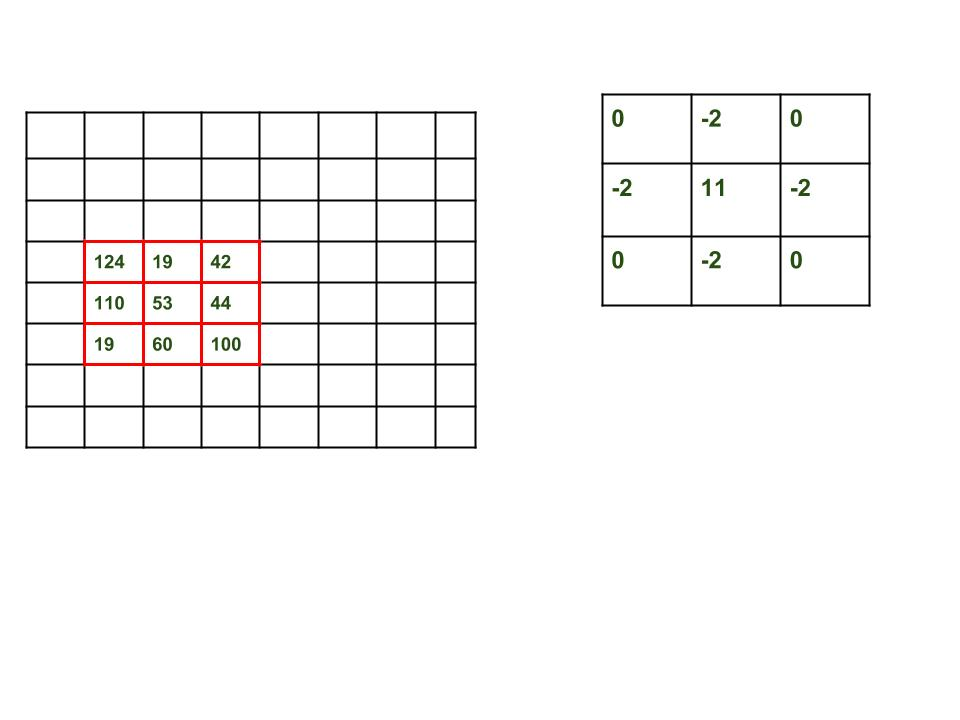Python Program to detect the edges of an image using OpenCV | Sobel edge detection method

• Difficulty Level : Medium
• Last Updated : 27 Dec, 2021

The following program detects the edges of frames in a livestream video content. The code will only compile in linux environment. Make sure that openCV is installed in your system before you run the program.

• Run the following command on your terminal to install it from the Ubuntu or Debian repository.

sudo apt-get install libopencv-dev python-opencv
•
• OR In order to download OpenCV from the official site run the following command:

bash install-opencv.sh

Principle behind Edge Detection

Edge detection involves mathematical methods to find points in an image where the brightness of pixel intensities changes distinctly.

• The first thing we are going to do is find the gradient of the grayscale image, allowing us to find edge-like regions in the x and y direction. The gradient is a multi-variable generalization of the derivative. While a derivative can be defined on functions of a single variable, for functions of several variables, the gradient takes its place.
• The gradient is a vector-valued function, as opposed to a derivative, which is scalar-valued. Like the derivative, the gradient represents the slope of the tangent of the graph of the function. More precisely, the gradient points in the direction of the greatest rate of increase of the function, and its magnitude is the slope of the graph in that direction.

Note: In computer vision, transitioning from black-to-white is considered a positive slope, whereas a transition from white-to-black is a negative slope.

Python

 # Python program to  Edge detection# using OpenCV in Python# using Sobel edge detection# and laplacian methodimport cv2import numpy as np #Capture livestream video content from camera 0cap = cv2.VideoCapture(0) while(1):     # Take each frame    _, frame = cap.read()         # Convert to HSV for simpler calculations    hsv = cv2.cvtColor(frame, cv2.COLOR_BGR2HSV)         # Calculation of Sobelx    sobelx = cv2.Sobel(frame,cv2.CV_64F,1,0,ksize=5)         # Calculation of Sobely    sobely = cv2.Sobel(frame,cv2.CV_64F,0,1,ksize=5)         # Calculation of Laplacian    laplacian = cv2.Laplacian(frame,cv2.CV_64F)         cv2.imshow('sobelx',sobelx)    cv2.imshow('sobely',sobely)    cv2.imshow('laplacian',laplacian)    k = cv2.waitKey(5) & 0xFF    if k == 27:        break cv2.destroyAllWindows() #release the framecap.release()

Calculation of the derivative of an image

A digital image is represented by a matrix that stores the RGB/BGR/HSV(whichever color space the image belongs to) value of each pixel in rows and columns.
The derivative of a matrix is calculated by an operator called the Laplacian. In order to calculate a Laplacian, you will need to calculate first two derivatives, called derivatives of Sobel, each of which takes into account the gradient variations in a certain direction: one horizontal, the other vertical.

• Horizontal Sobel derivative (Sobel x): It is obtained through the convolution of the image with a matrix called kernel which has always odd size. The kernel with size 3 is the simplest case.
• Vertical Sobel derivative (Sobel y): It is obtained through the convolution of the image with a matrix called kernel which has always odd size. The kernel with size 3 is the simplest case.
• Convolution is calculated by the following method: Image represents the original image matrix and filter is the kernel matrix.• Factor = 11 – 2- 2- 2- 2- 2 = 3
Offset = 0
Weighted Sum = 124*0 + 19*(-2) + 110*(-2) + 53*11 + 44*(-2) + 19*0 + 60*(-2) + 100*0 = 117
O[4,2] = (117/3) + 0 = 39
So in the end to get the Laplacian (approximation) we will need to combine the two previous results (Sobelx and Sobely) and store it in laplacian.

Parameters:

• cv2.Sobel(): The function cv2.Sobel(frame,cv2.CV_64F,1,0,ksize=5) can be written as
cv2.Sobel(original_image,ddepth,xorder,yorder,kernelsize)
• where the first parameter is the original image, the second parameter is the depth of the destination image. When ddepth=-1/CV_64F, the destination image will have the same depth as the source. The third parameter is the order of the derivative x. The fourth parameter is the order of the derivative y. While calculating Sobelx we will set xorder as 1 and yorder as 0 whereas while calculating Sobely, the case will be reversed. The last parameter is the size of the extended Sobel kernel; it must be 1, 3, 5, or 7.
• cv2.Laplacian: In the function
cv2.Laplacian(frame,cv2.CV_64F)
• the first parameter is the original image and the second parameter is the depth of the destination image.When depth=-1/CV_64F, the destination image will have the same depth as the source.

Edge Detection Applications

• Reduce unnecessary information in an image while preserving the structure of image.
• Extract important features of image like curves, corners and lines.
• Recognizes objects, boundaries and segmentation.
• Plays a major role in computer vision and recognition

Related Article: Edge Detection using Canny edge detection method